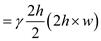# Consider a 4-m-long, 4-m-wide, and 1.5 aboveground swimming pool that is filled with water to their rim. (a) Determine the hydrostatic force on each wall and distance of the line of action of this force from the (b) If the height of the walls of the pool is doubled and pool is filled, will the hydrostatic force on each wall double or quadruple? Why?

Question-AnswerCategory: Fluid MechanicsConsider a 4-m-long, 4-m-wide, and 1.5 aboveground swimming pool that is filled with water to their rim. (a) Determine the hydrostatic force on each wall and distance of the line of action of this force from the (b) If the height of the walls of the pool is doubled and pool is filled, will the hydrostatic force on each wall double or quadruple? Why?

Consider a 4-m-long, 4-m-wide, and 1.5 aboveground swimming pool that is filled with water to their rim. (a) Determine the hydrostatic force on each wall and distance of the line of action of this force from the (b) If the height of the walls of the pool is doubled and pool is filled, will the hydrostatic force on each wall double or quadruple? Why?

Step: 1

Given:
Length of swimming pool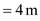Width of swimming poolHeight of swimming poolStep: 2

Specific weight of water,Area,The distance from free surface of water to centroid of the wall:Step: 3

(a)
Hydrostatic force on the wall: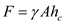Thus the hydrostatic force on each wall is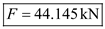Step: 4

Distance of line of action from the ground: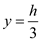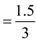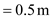Thus the distance of line of action from the ground is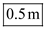.

Step: 5

(b)
Hydrostatic force on the wall when the height of the pool is doubled: